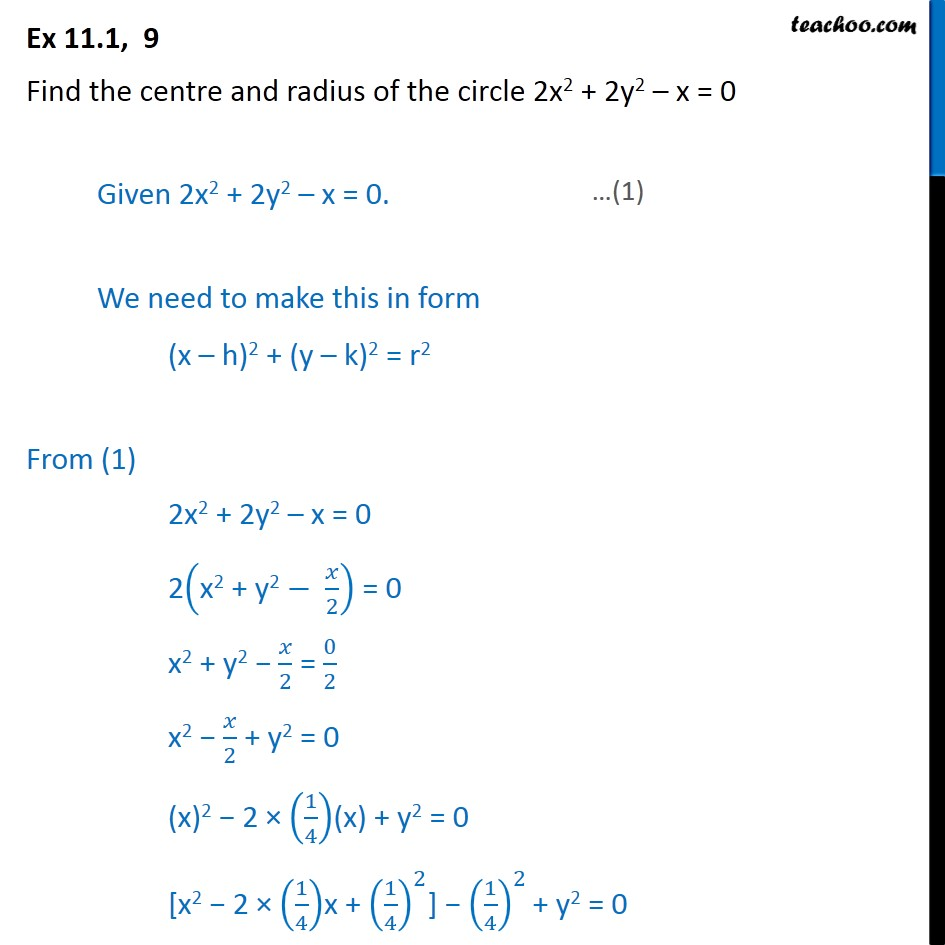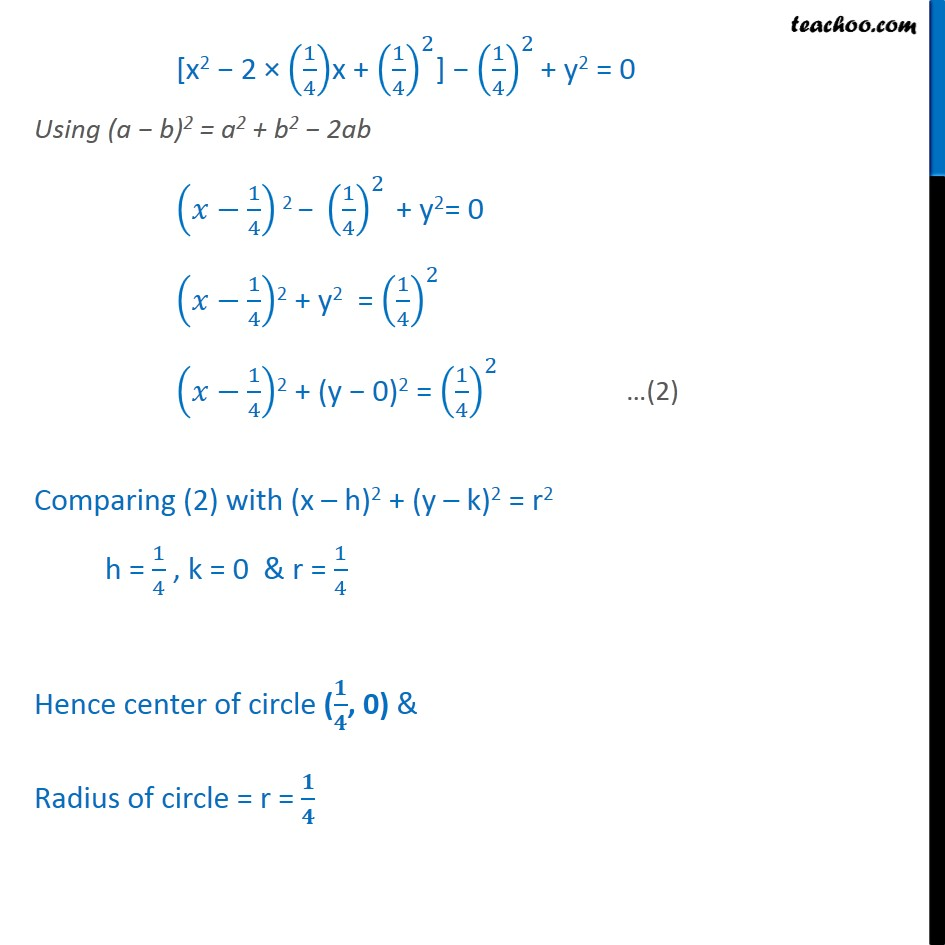1. Chapter 11 Class 11 Conic Sections
2. Serial order wise
3. Ex 11.1

Transcript

Ex 11.1, 9 Find the centre and radius of the circle 2x2 + 2y2 – x = 0 Given 2x2 + 2y2 – x = 0. We need to make this in form (x – h)2 + (y – k)2 = r2 From (1) 2x2 + 2y2 – x = 0 2﷐x2 + y2− ﷐𝑥﷮2﷯﷯ = 0 x2 + y2 − ﷐𝑥﷮2﷯ = ﷐0﷮2﷯ x2 − ﷐𝑥﷮2﷯ + y2 = 0 (x)2 − 2 × ﷐﷐1﷮4﷯﷯(x) + y2 = 0 [x2 − 2 × ﷐﷐1﷮4﷯﷯x + ﷐﷐﷐1﷮4﷯﷯﷮2﷯] − ﷐﷐﷐1﷮4﷯﷯﷮2﷯ + y2 = 0 [x2 − 2 × ﷐﷐1﷮4﷯﷯x + ﷐﷐﷐1﷮4﷯﷯﷮2﷯] − ﷐﷐﷐1﷮4﷯﷯﷮2﷯ + y2 = 0 Using (a − b)2 = a2 + b2 − 2ab ﷐𝑥−﷐1﷮4﷯﷯2 − ﷐﷐﷐1﷮4﷯﷯﷮2﷯ + y2= 0 ﷐𝑥−﷐1﷮4﷯﷯2 + y2 = ﷐﷐﷐1﷮4﷯﷯﷮2﷯ ﷐𝑥−﷐1﷮4﷯﷯2 + (y − 0)2 = ﷐﷐﷐1﷮4﷯﷯﷮2﷯ Comparing (2) with (x – h)2 + (y – k)2 = r2 h = ﷐1﷮4﷯ , k = 0 & r = ﷐1﷮4﷯ Hence center of circle (﷐𝟏﷮𝟒﷯, 0) & Radius of circle = r = ﷐𝟏﷮𝟒﷯

Ex 11.1

About the AuthorDavneet Singh
Davneet Singh is a graduate from Indian Institute of Technology, Kanpur. He has been teaching from the past 9 years. He provides courses for Maths and Science at Teachoo.